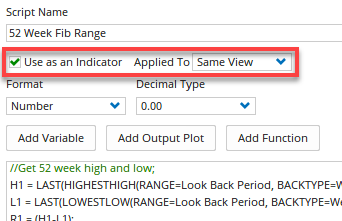# Custom Scripted Tool - 52 week Fibonacci Levels

Author: Optuma Team Last updated: Jan 31, 2023 15:23

## Overview

Any script formula applied to a Show Bar, Show Plot or Show View can be saved as your own tool and added to your toolbox. For example, the following script can be used in a Show Plot to automatically calculate the Fibonacci levels between the 52 week high and low using multiple Plot functions:

``````//Get 52 week high and low;
H1 = LAST(HIGHESTHIGH(RANGE=Look Back Period, BACKTYPE=Weeks, BARS=52, INCBAR=True));
L1 = LAST(LOWESTLOW(RANGE=Look Back Period, BACKTYPE=Weeks, BARS=52, INCBAR=True));
//Calc range;
R1 = (H1-L1);

//Calc Fib levels;
Plot1 = H1;
Plot2 = L1 + (R1 * 0.618);
Plot3 = L1 + (R1 * 0.5);
Plot4 = L1 + (R1 * 0.382);
Plot5 = L1;

//Set line styles;
Plot1.Colour = Green;
Plot1.Linestyle = Dash;
Plot1.LineWidth = 2;

Plot2.Colour = Blue;
Plot2.Linestyle = Dash;

Plot3.Colour = Red;
Plot3.Linestyle = Dash;
Plot3.LineWidth = 2;

Plot4.Colour = Blue;
Plot4.Linestyle = Dash;

Plot5.Colour = Green;
Plot5.Linestyle = Dash;
Plot5.LineWidth = 2;
``````

To save as an indicator and add it to your toolbox, click on the Use as an Indicator box, and apply it to the Same View so that it appears on the price chart.Once saved, apply the tool as per any other tool from your toolbox and the Fibonacci levels will automatically show on the screen - and update automatically as the high/low range changes.